# Easy Way To Calculate Poker Odds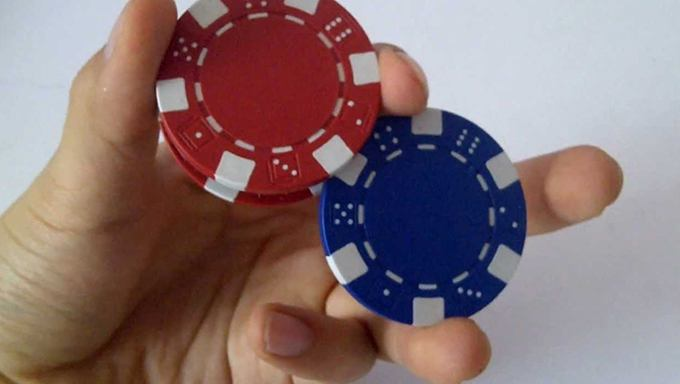If you don’t have a poker odds table or can’t use it, there is an easy way to calculate poker odds. You can use this calculation method in live poker.

Let’s take an example:. You hold in your hands: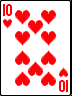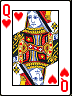The flop fell: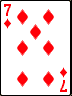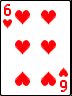And the question: What are your poker odds caught in the Color Combination next round?

Solution:

First we count the outs: unknown cards that will help us to match the color combination. There are 9 of them.

Use the following rule: Multiply Known Outs by 2 and Convert by Percent if you want to see poker odds on a single card, and multiply by 4, Percent Outs to see by poker on two cards.

So in our case, we multiply 9 by 2 and convert it into a percentage. We get 18%. This means that 18% of the times will show you the cards that will help you and 82% of the times will show you the cards that will not help you. This odds ours out: 82: 18 = 4.5: 1. This answer is 4.5: 1. This is not the exact result as given in the table but close to the truth. However, this is a great way to easily calculate odds when you don’t have a table next to odds.

And if you want to know the odds on the two cards, you multiply 9 by 4 and convert it to a percentage. We get 36%. This means that 36% of the time, the cards that will help you open, and 64% of the times, the cards that will not help you. That’s the odds of our coming out: 64: 36 = 1.7: 1. That’s the answer is 1.7: 1. That’s not the exact result, but it’s also close to the truth.